• #卡方分箱代码 def ChiMerge(train_new, feature, chuShiQieXiang, xiangShu): d1 = pd.DataFrame({'X':train_new[feature], 'Y':train_new.iloc[:,0], feature:pd.qcut(train_new[feature],chuShiQieXiang)}) d2 ...
#卡方分箱代码 def ChiMerge(train_new, feature, chuShiQieXiang, xiangShu):     d1 = pd.DataFrame({'X':train_new[feature], 'Y':train_new.iloc[:,0], feature:pd.qcut(train_new[feature],chuShiQieXiang)})     d2 = d1.groupby(feature,as_index=True)     d3=pd.concat([d2.sum()[['Y']],d2[['Y']].count()-d2.sum()[['Y']]],axis=1)     d3.columns=['bad','good']     d3['index']=d3.index     d3.index = range(len(d3))     while(len(d3)>xiangShu):         X2=[]         for i in range (0,len(d3)-1):             chi2,p,dof,expect=stats.chi2_contingency(d3.iloc[i:i+2,0:2])             X2.append(chi2)         ind=X2.index(min(X2))         chaR=d3.iloc[ind,0:2]+d3.iloc[ind+1,0:2]         charu=pd.DataFrame({'bad':chaR['bad'],'good':chaR['good'],'index':[pd.Interval(d3.iloc[ind]['index'].left,d3.iloc[ind+1]['index'].right)]})         d3.drop(d3.iloc[ind:ind+2,:].index,inplace=True)         heBing=pd.concat([d3,charu])         heBing=heBing.sort_values('index')         heBing.index = range(len(heBing))         d3=heBing     d3.columns=['bad','good',feature]     return d3
展开全文• 卡方分箱算法ChiMerge卡方分箱算法ChiMerge卡方分箱原理阐述，论文
• 1、实现了二分类的卡方分箱； 2、实现了最大分组限定停止条件，和最小阈值限定停止条件； 问题，还不太清楚，后续补充。 1、自由度k,如何来确定，卡方阈值的自由度为 分箱数-1，显著性水平可以取10%，5%或1% 算法...
• 1、实现了二分类的卡方分箱； 2、实现了最大分组限定停止条件，和最小阈值限定停止条件； 问题，还不太清楚，后续补充。 1、自由度k,如何来确定，卡方阈值的自由度为 分箱数-1，显著性水平可以取10%，5%或1% 算法...
解决的问题： 1、实现了二分类的卡方分箱； 2、实现了最大分组限定停止条件，和最小阈值限定停止条件；
问题，还不太清楚，后续补充。 1、自由度k,如何来确定，卡方阈值的自由度为 分箱数-1，显著性水平可以取10%，5%或1%
算法扩展： 1、卡方分箱除了用阈值来做约束条件，还可以进一步的加入分箱数约束，以及最小箱占比，坏人率约束等。 2、需要实现更多分类的卡方分箱算法；

具体代码如下：
# -*- coding: utf-8 -*-
"""
Created on Wed Nov 28 16:54:58 2018

@author: wolfly_fu

解决的问题：
1、实现了二分类的卡方分箱
2、实现了最大分组限定停止条件，和最小阈值限定停止条件；

问题，
1、自由度k,如何来确定？

算法扩展：
1、卡方分箱除了用阈值来做约束条件，还可以进一步的加入分箱数约束，以及最小箱占比，坏人率约束等。
2、需要实现更多分类的卡方分箱算法

"""

import pandas as pd
import numpy as np
from scipy.stats import chi2

#导入数据

#计算卡方统计量
def cal_chi2(input_df, var_name, Y_name):  ##二分类，，计算每个变量值的卡方统计量
'''
df = input_df[[var_name, Y_name]]
var_values = sorted(list(set(df[var_name])))
Y_values = sorted(list(set(df[Y_name])))
#用循环的方式填充
chi2_result = pd.DataFrame(index=var_values, columns=Y_values)
for var_value in var_values:
for Y_value in Y_values:
chi2_result.loc[var_value][Y_value] = \
df[(df[var_name]==var_value)&(df[Y_name]==Y_value)][var_name].count()
'''
input_df = input_df[[var_name, Y_name]]   #取数据
all_cnt = input_df[Y_name].count() #样本总数
all_0_cnt = input_df[input_df[Y_name] == 0].shape # 二分类的样本数量
all_1_cnt = input_df[input_df[Y_name] == 1].shape
expect_0_ratio = all_0_cnt * 1.0 / all_cnt #样本分类比例
expect_1_ratio = all_1_cnt * 1.0 / all_cnt

#对变量的每个值计算实际个数，期望个数，卡方统计量
var_values = sorted(list(set(input_df[var_name])))
actual_0_cnt = []        #  actual_0  该值，类别为0的数量
actual_1_cnt = []        #  actual_1  该值，类别为1的数量
actual_all_cnt = []
expect_0_cnt = []       # expect_0 类别0 的卡方值
expect_1_cnt = []       # expect_1 类别1 的卡方值
chi2_value = []         # chi2_value 该组的卡方值

for value in var_values:
actual_0 = input_df[(input_df[var_name]==value)&(input_df[Y_name]==0)].shape #该值，类别为0的数量
actual_1 = input_df[(input_df[var_name]==value)&(input_df[Y_name]==1)].shape
actual_all = actual_0 + actual_1 #总数
expect_0 = actual_all * expect_0_ratio #类别0 的 期望频率
expect_1 = actual_all * expect_1_ratio

chi2_0 = (expect_0 - actual_0)**2 / expect_0 #类别0 的卡方值
chi2_1 = (expect_1 - actual_1)**2 / expect_1

actual_0_cnt.append(actual_0)  #样本为0的，该值的数量
actual_1_cnt.append(actual_1)

actual_all_cnt.append(actual_all) #改组的总样本数
expect_0_cnt.append(expect_0)  #类别0 的 期望频率
expect_1_cnt.append(expect_1)

chi2_value.append(chi2_0 + chi2_1) #改变量值的卡方值

chi2_result = pd.DataFrame({'actual_0':actual_0_cnt, 'actual_1':actual_1_cnt, 'expect_0':expect_0_cnt, \
'expect_1':expect_1_cnt, 'chi2_value':chi2_value, var_name+'_start':var_values, \
var_name+'_end':var_values}, \
columns=[var_name+'_start', var_name+'_end', 'actual_0', 'actual_1', 'expect_0', 'expect_1', 'chi2_value'])

return chi2_result, var_name

#定义合并区间的方法
def merge_area(chi2_result, var_name, idx, merge_idx):
#按照idx和merge_idx执行合并
chi2_result.ix[idx, 'actual_0'] = chi2_result.ix[idx, 'actual_0'] + chi2_result.ix[merge_idx, 'actual_0']
chi2_result.ix[idx, 'actual_1'] = chi2_result.ix[idx, 'actual_1'] + chi2_result.ix[merge_idx, 'actual_1']
chi2_result.ix[idx, 'expect_0'] = chi2_result.ix[idx, 'expect_0'] + chi2_result.ix[merge_idx, 'expect_0']
chi2_result.ix[idx, 'expect_1'] = chi2_result.ix[idx, 'expect_1'] + chi2_result.ix[merge_idx, 'expect_1']
chi2_0 = (chi2_result.ix[idx, 'expect_0'] - chi2_result.ix[idx, 'actual_0'])**2 / chi2_result.ix[idx, 'expect_0']
chi2_1 = (chi2_result.ix[idx, 'expect_1'] - chi2_result.ix[idx, 'actual_1'])**2 / chi2_result.ix[idx, 'expect_1']

chi2_result.ix[idx, 'chi2_value'] = chi2_0 + chi2_1     #计算卡方值

#调整每个区间的起始值
if idx < merge_idx:
chi2_result.ix[idx, var_name+'_end'] = chi2_result.ix[merge_idx, var_name+'_end'] #向后扩大范围
else:
chi2_result.ix[idx, var_name+'_start'] = chi2_result.ix[merge_idx, var_name+'_start']  ##，向前扩大范围

chi2_result = chi2_result.drop([merge_idx]) #删掉行
chi2_result = chi2_result.reset_index(drop=True)

return chi2_result

#自动进行分箱，使用最大区间限制
def chiMerge_maxInterval(chi2_result, var_name, max_interval=5):  #最大分箱数 为  5
groups = chi2_result.shape #各组的卡方值，数量
while groups > max_interval:
min_idx = chi2_result[chi2_result['chi2_value']==chi2_result['chi2_value'].min()].index.tolist() #寻找最小的卡方值
if min_idx == 0:
chi2_result = merge_area(chi2_result, var_name, min_idx, min_idx+1)  #合并1和2组
elif min_idx == groups-1:
chi2_result = merge_area(chi2_result, var_name, min_idx, min_idx-1)

else: #寻找左右两边更小的卡方组
if chi2_result.loc[min_idx-1, 'chi2_value'] > chi2_result.loc[min_idx+1, 'chi2_value']:
chi2_result = merge_area(chi2_result, var_name, min_idx, min_idx+1)
else:
chi2_result = merge_area(chi2_result, var_name, min_idx, min_idx-1)
groups = chi2_result.shape

return chi2_result

def chiMerge_minChiSquare(chi2_result, var_name): #(chi_result, maxInterval=5):
'''
卡方分箱合并--卡方阈值法，，同时限制，最大组为6组，，可以去掉
'''
threshold = get_chiSquare_distribution(4, 0.1)
min_chiSquare = chi2_result['chi2_value'].min()
#min_chiSquare = chi_result['chi_square'].min()
group_cnt = len(chi2_result)
# 如果变量区间的最小卡方值小于阈值，则继续合并直到最小值大于等于阈值
while(min_chiSquare < threshold and group_cnt > 6):
min_idx = chi2_result[chi2_result['chi2_value']==chi2_result['chi2_value'].min()].index.tolist() #寻找最小的卡方值
#min_index = chi_result[chi_result['chi_square']==chi_result['chi_square'].min()].index.tolist()
# 如果分箱区间在最前,则向下合并
if min_idx == 0:
chi2_result = merge_area(chi2_result, var_name, min_idx, min_idx+1)  #合并1和2组
elif min_idx == group_cnt -1:
chi2_result = merge_area(chi2_result, var_name, min_idx, min_idx-1)

else: #寻找左右两边更小的卡方组
if chi2_result.loc[min_idx-1, 'chi2_value'] > chi2_result.loc[min_idx+1, 'chi2_value']:
chi2_result = merge_area(chi2_result, var_name, min_idx, min_idx+1)
else:
chi2_result = merge_area(chi2_result, var_name, min_idx, min_idx-1)

min_chiSquare = chi2_result['chi2_value'].min()
group_cnt = len(chi2_result)

return chi2_result

#分箱主体部分包括两种分箱方法的主体函数，其中merge_chiSquare()是对区间进行合并，
#get_chiSquare_distribution()是根据自由度和置信度得到卡方阈值。我在这里设置的是自由度为4
#，置信度为10%。两个自定义函数如下

def get_chiSquare_distribution(dfree=4, cf=0.1):
'''
根据自由度和置信度得到卡方分布和阈值
dfree:自由度k= (行数-1)*(列数-1)，默认为4     #问题，自由度k,如何来确定？
cf:显著性水平，默认10%
'''
percents = [ 0.95, 0.90, 0.5,0.1, 0.05, 0.025, 0.01, 0.005]
df = pd.DataFrame(np.array([chi2.isf(percents, df=i) for i in range(1, 30)]))
df.columns = percents
df.index = df.index+1
# 显示小数点后面数字
pd.set_option('precision', 3)
return df.loc[dfree, cf]
参考-感谢：
1、 https://blog.csdn.net/weixin_42097808/article/details/80494939
2、https://blog.csdn.net/wyl2289/article/details/81981145
3、http://www.sohu.com/a/224569101_793685

展开全文• ## python实现卡方分箱Chi-Merge

万次阅读 多人点赞 2018-05-29 14:25:25
卡方分箱是依赖于卡方检验的分箱方法，在统计指标上选择卡方统计量（chi-Square）进行判别，分箱的基本思想是判断相邻的两个区间是否有分布差异，基于卡方统计量的结果进行自下而上的合并，直到满足分箱的限制条件...
卡方分箱是依赖于卡方检验的分箱方法，在统计指标上选择卡方统计量（chi-Square）进行判别，分箱的基本思想是判断相邻的两个区间是否有分布差异，基于卡方统计量的结果进行自下而上的合并，直到满足分箱的限制条件为止。
卡方分箱的实现步骤：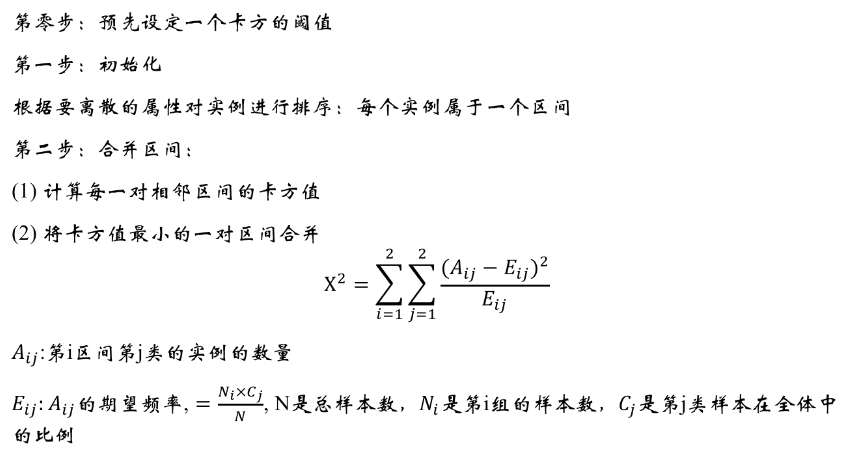卡方统计量衡量了区间内样本的频数分布与整体样本的频数分布的差异性，在做分箱处理时可以使用两种限制条件：
（1）分箱个数：限制最终的分箱个数结果，每次将样本中具有最小卡方值的区间与相邻的最小卡方区间进行合并，直到分箱个数达到限制条件为止。
（2）卡方阈值：根据自由度和显著性水平得到对应的卡方阈值，如果分箱的各区间最小卡方值小于卡方阈值，则继续合并，直到最小卡方值超过设定阈值为止。
在铺上代码前再补充两点，
1、由于卡方分箱是思想是相邻区间合并，在初始化时对变量属性需先进行排序，要注意名义变量的排序顺序
2、卡方阈值的自由度为 分箱数-1，显著性水平可以取10%，5%或1%
python代码实现：举例：卡方对二分类问题进行变量分箱
import pandas as pd
import numpy as np
from scipy.stats import chi2

def calc_chiSquare(sampleSet, feature, target):
'''
计算某个特征每种属性值的卡方统计量
params:
sampleSet: 样本集
feature: 目标特征
target: 目标Y值 (0或1) Y值为二分类变量
return:
卡方统计量dataframe
feature: 特征名称
act_target_cnt: 实际坏样本数
expected_target_cnt：期望坏样本数
chi_square：卡方统计量
'''
# 计算样本期望频率
target_cnt = sampleSet[target].sum()
sample_cnt = len(sampleSet[target])
expected_ratio = target_cnt * 1.0/sample_cnt
# 对变量按属性值从大到小排序
df = sampleSet[[feature, target]]
col_value = list(set(df[feature]))
# 计算每一个属性值对应的卡方统计量等信息
chi_list = []; target_list = []; expected_target_list = []
for value in col_value:
df_target_cnt = df.loc[df[feature] == value, target].sum()
df_cnt = len(df.loc[df[feature] == value, target])
expected_target_cnt = df_cnt * expected_ratio
chi_square = (df_target_cnt - expected_target_cnt)**2 / expected_target_cnt
chi_list.append(chi_square)
target_list.append(df_target_cnt)
expected_target_list.append(expected_target_cnt)
# 结果输出到dataframe, 对应字段为特征属性值, 卡方统计量, 实际坏样本量, 期望坏样本量
chi_stats = pd.DataFrame({feature:col_value, 'chi_square':chi_list,
'act_target_cnt':target_list, 'expected_target_cnt':expected_target_list})
return chi_stats[[feature, 'act_target_cnt', 'expected_target_cnt', 'chi_square']]

var 表示需要分箱的变量，函数返回卡方统计结果，包括样本实例区间，卡方统计量，响应频率和期望响应频率。
def chiMerge_maxInterval(chi_stats, feature, maxInterval=5):
'''
卡方分箱合并--最大区间限制法
params:
chi_stats: 卡方统计量dataframe
feature: 目标特征
maxInterval：最大分箱数阈值
return:
卡方合并结果dataframe, 特征分割split_list
'''
group_cnt = len(chi_stats)
split_list = [chi_stats[feature].min()]
# 如果变量区间超过最大分箱限制，则根据合并原则进行合并
while(group_cnt > maxInterval):
min_index = chi_stats[chi_stats['chi_square']==chi_stats['chi_square'].min()].index.tolist()
# 如果分箱区间在最前,则向下合并
if min_index == 0:
chi_stats = merge_chiSquare(chi_stats, min_index+1, min_index)
# 如果分箱区间在最后，则向上合并
elif min_index == group_cnt-1:
chi_stats = merge_chiSquare(chi_stats, min_index-1, min_index)
# 如果分箱区间在中间，则判断与其相邻的最小卡方的区间，然后进行合并
else:
if chi_stats.loc[min_index-1, 'chi_square'] > chi_stats.loc[min_index+1, 'chi_square']:
chi_stats = merge_chiSquare(chi_stats, min_index, min_index+1)
else:
chi_stats = merge_chiSquare(chi_stats, min_index-1, min_index)
group_cnt = len(chi_stats)
chiMerge_result = chi_stats
split_list.extend(chiMerge_result[feature].tolist())
return chiMerge_result, split_list

def chiMerge_minChiSquare(chi_stats, feature, dfree=4, cf=0.1, maxInterval=5):
'''
卡方分箱合并--卡方阈值法
params:
chi_stats: 卡方统计量dataframe
feature: 目标特征
maxInterval: 最大分箱数阈值, default 5
dfree: 自由度, 最大分箱数-1, default 4
cf: 显著性水平, default 10%
return:
卡方合并结果dataframe, 特征分割split_list
'''
threshold = get_chiSquare_distuibution(dfree, cf)
min_chiSquare = chi_stats['chi_square'].min()
group_cnt = len(chi_stats)
split_list = [chi_stats[feature].min()]
# 如果变量区间的最小卡方值小于阈值，则继续合并直到最小值大于等于阈值
while(min_chiSquare < threshold and group_cnt > maxInterval):
min_index = chi_stats[chi_stats['chi_square']==chi_stats['chi_square'].min()].index.tolist()
# 如果分箱区间在最前,则向下合并
if min_index == 0:
chi_stats = merge_chiSquare(chi_stats, min_index+1, min_index)
# 如果分箱区间在最后，则向上合并
elif min_index == group_cnt-1:
chi_stats = merge_chiSquare(chi_stats, min_index-1, min_index)
# 如果分箱区间在中间，则判断与其相邻的最小卡方的区间，然后进行合并
else:
if chi_stats.loc[min_index-1, 'chi_square'] > chi_stats.loc[min_index+1, 'chi_square']:
chi_stats = merge_chiSquare(chi_stats, min_index, min_index+1)
else:
chi_stats = merge_chiSquare(chi_stats, min_index-1, min_index)
min_chiSquare = chi_stats['chi_square'].min()
group_cnt = len(chi_stats)
chiMerge_result = chi_stats
split_list.extend(chiMerge_result[feature].tolist())
return chiMerge_result, split_list
分箱主体部分包括两种分箱方法的主体函数，其中merge_chiSquare()是对区间进行合并，get_chiSquare_distribution()是根据自由度和置信度得到卡方阈值。我在这里设置的是自由度为4，置信度为10%。两个自定义函数如下
def get_chiSquare_distuibution(dfree=4, cf=0.1):
'''
根据自由度和置信度得到卡方分布和阈值
params:
dfree: 自由度, 最大分箱数-1, default 4
cf: 显著性水平, default 10%
return:
卡方阈值
'''
percents = [0.95, 0.90, 0.5, 0.1, 0.05, 0.025, 0.01, 0.005]
df = pd.DataFrame(np.array([chi2.isf(percents, df=i) for i in range(1, 30)]))
df.columns = percents
df.index = df.index+1
# 显示小数点后面数字
pd.set_option('precision', 3)
return df.loc[dfree, cf]
def merge_chiSquare(chi_result, index, mergeIndex, a = 'expected_target_cnt',
b = 'act_target_cnt', c = 'chi_square'):
'''
params:
chi_result: 待合并卡方数据集
index: 合并后的序列号
mergeIndex: 需合并的区间序号
a, b, c: 指定合并字段
return:
分箱合并后的卡方dataframe
'''
chi_result.loc[mergeIndex, a] = chi_result.loc[mergeIndex, a] + chi_result.loc[index, a]
chi_result.loc[mergeIndex, b] = chi_result.loc[mergeIndex, b] + chi_result.loc[index, b]
chi_result.loc[mergeIndex, c] = (chi_result.loc[mergeIndex, b] - chi_result.loc[mergeIndex, a])**2 /chi_result.loc[mergeIndex, a]
chi_result = chi_result.drop([index])
chi_result = chi_result.reset_index(drop=True)
return chi_result 
补充个例子，特征是identity_age， Y值是target(0, 1)
根据卡方分箱结果得到最后的分割点list是[1, 19, 21, 23, 24, 26, 59]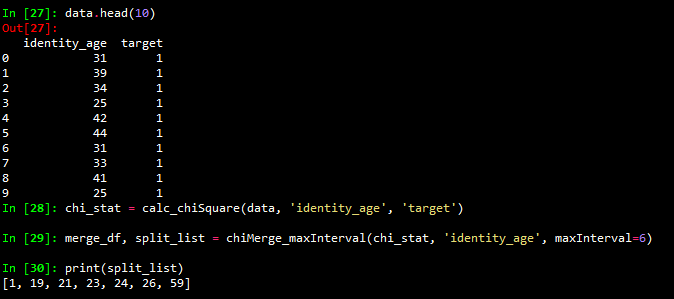展开全文• 特征离散化 之 卡方分箱 离散特征在数据挖掘的过程中具有重要作用，因此特征离散化是构建特征工程的一个很常见、也很重要的环节。 最近做项目需要用到卡方分箱实现特征的离散化，发现这么经典的功能python竟然...
特征离散化（一） 之 卡方分箱 特征离散化（二） 之 Chi2分箱 特征离散化（三） 之 最小熵分箱 特征离散化（四） 之 bestKS分箱 特征离散化（五） 之 评分卡最优分箱
离散特征在数据挖掘的过程中具有重要作用，因此特征离散化是构建特征工程的一个很常见、也很重要的环节。
卡方分箱作为最经典的离散化方法之一，最近做项目需要用到时，却发现这么经典的功能python竟然没有官方的封装库。找了许多资料，感觉讲的都比较杂（一会chiMerge，一会chi2，一会单调性检验 O__O”… ），看的怀疑人生。最后实在不得已，只能翻出原论文 ChiMerge: Discretization of Numeric Attributes 出来拜读一下。
看完论文后发现最原始的卡方分箱思想还是挺简单的，只是网上很多资料讲的层次不清晰，让很多初学者看昏了头。因此写篇博客记录复现时的一些想法和踩过的坑。
1. 分箱
首先，介绍一下什么是分箱。分箱是将连续变量离散化，多状态的离散变量合并成少状态的过程。这句话里包含两个要点，第一点：分箱的对象可以是连续变量，也可以是离散变量。第二点：分箱的目的是将变量的可取值变少（更便于分析）。目前主流的分箱方法可以分为两大类：1）自底向上的基于合并（merge）机制的方法，如卡方分箱；2）自上向下的基于分割（split）机制的方法，如基于决策树的分箱、bestKS分箱。后续，博主将一一开设博客介绍这些方法，敬请期待。
2. 卡方分箱 之 ChiMerge
前面说过，卡方分箱是典型的基于合并机制的自底向上离散化方法。其基于如下假设：如果两个相邻的区间具有非常类似的类分布，则这两个区间可以合并；否则，它们应当保持分开。此处衡量分布相似性的指标就是卡方值。卡方值越低，类分布的相似度越高。
因此，ChiMerge分箱的主要思想归结为一句话就是:将具有最小卡方值的相邻区间合并在一起,直到满足确定的停止准则。其通用流程如下：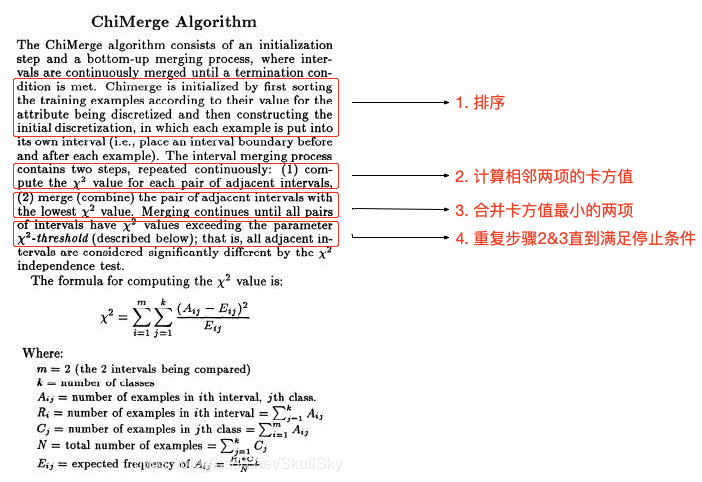这里面包含四个关键点： 1. 离散变量如何排序：大部分关于卡方分箱的介绍都是针对连续变量，对于离散变量，应该如何处理 2. 卡方值计算（坑最深的地方）：如何计算相邻两项的卡方值 3. 区间合并：如何合并卡方值最小的两项 4. 停止条件：何时结束循环，停止分箱 下面我们将具体讨论这些问题。
2.1. 排序
卡方分箱的第一步即对数据排序。对于连续变量，直接根据变量数值大小排序即可。对于离散变量，由于取值不存在大小关系，无法直接排序。这里一般采用的排序依据是：正例样本的比例，即待分箱变量每个取值中正例样本的比重，对应代码中的pos_ratio属性。
那么具体如何排序呢？我们前面提到过，卡方分箱是基于合并机制的离散化方法。因此，初始的分箱状态为：将待分箱变量的每个取值视为一个单独的箱体，后续分箱的目的就是将这些箱体合并为若干个箱体。首先，我们统计待分箱变量的可选取值，以及各个取值的正负样本数量（count)，然后判断变量类型确定排序依据。
代码如下：其中，var_name_bf 表示需要分箱的变量，函数返回排序后的待分箱变量的统计分布，包括样本取值，正例样本，负例样本。
def dsct_init(data, var_name_bf, var_name_target, feature_type):
"""
特征离散化节点初始化：统计各取值的正负样本分布 [正例样本个数，负例样本个数] 并排序
:param data: DataFrame 输入数据
:param var_name_bf: str 待分箱变量
:param var_name_target: str 标签变量（y)
:param feature_type: 特征的类型：0（连续） 1（离散）
:return: DataFrame 排好序的各组中正负样本分布 count
"""
# 统计待离散化变量的取值类型（string or digits)
data_type = data[var_name_bf].apply(lambda x: type(x)).unique()
var_type = True if str in data_type else False # 实际取值的类型：false(数字） true(字符）

# 是否需要根据正例样本比重排序，True：需要，False：不需要
#                   0（连续）    1（离散）
#     false（数字）    0              0（离散有序）
#     true（字符）     ×             1（离散无序）
if feature_type == var_type:
ratio_indicator = var_type
elif feature_type == 1:
ratio_indicator = 0
print("特征%s为离散有序数据，按照取值大小排序！" % (var_name_bf))
elif feature_type == 0:
exit(code="特征%s的类型为连续型，与其实际取值（%s）型不一致，请重新定义特征类型！！！" % (var_name_bf, data_type))

# 统计各分箱（group）内正负样本分布[累计样本个数，正例样本个数，负例样本个数]
count = pd.crosstab(data[var_name_bf], data[var_name_target])
total = count.sum(axis=1)

# 排序：离散变量按照pos_ratio排序，连续变量按照index排序
if ratio_indicator:
count['pos_ratio'] = count.sum(axis=1) * 1.0 / total #计算正例比例
count = count.sort_values('pos_ratio') #离散变量按照pos_ratio排序
count = count.drop(columns = ['pos_ratio'])
else:
count = count.sort_index() # 连续变量按照index排序
return count, ratio_indicator

需要注意的是，如果待分箱变量为离散变量，该方法只能使用于二分类模型。因为计算pos_ratio时，要求

y

∈

[

0

,

1

]

y \in [0,1]

。当然，这里可以根据个人需要调整pos_ratio的计算方式，以适应多分类问题。
2.2. 卡方值计算
大多数介绍卡方分箱的文章都没有具体解释相邻区间的卡方值如何计算。在原论文中对于卡方值计算也比较简略，这部分将着重讨论这一内容。首先，给出卡方值的计算公式，如下图所示（左边：数据，右边：对应的卡方值计算公式）。从公式来看，卡方值的计算其实并不复杂。对于四联表中的每一项，分别计算每一项的期望值（分母部分），并计算实际值与期望值之间的差异。不太了解的同学可以参考这篇博客卡方分箱中卡方值的计算。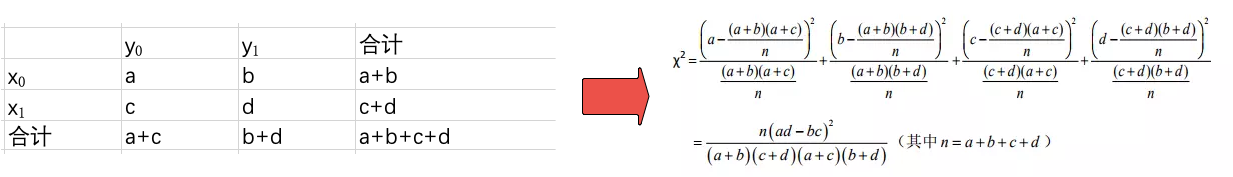然后，给出原文中关于卡方分箱方法中卡方值计算的介绍，内容如下（左边为原文，右边是从某篇中文文献中截取出来的中文解释）。对于卡方分箱中卡方值的计算，这里有个需要注意的地方：重点观察下标

i

i

，

j

j

的取值变化。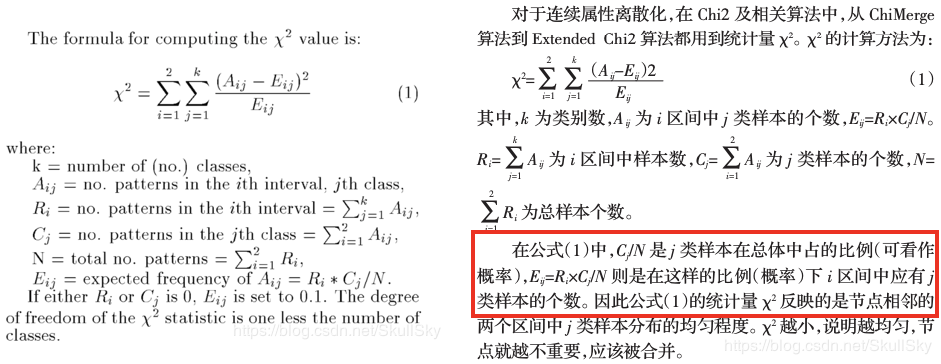假设我们的待分箱矩阵A如下图右边所示，为了方便表示，矩阵中的数值用字母a,b,…表示。

R

i

R_i

和

C

j

C_j

分别是第

i

i

行数据的和以及第

j

j

列数据的和，其中，

j

∈

[

0

,

k

]

j \in[0, k]

,

k

k

是类别数（这里y只有两个取值，所以

k

=

2

k=2

），

i

∈

[

0

,

z

]

i \in[0, z]

，

z

z

是样本数。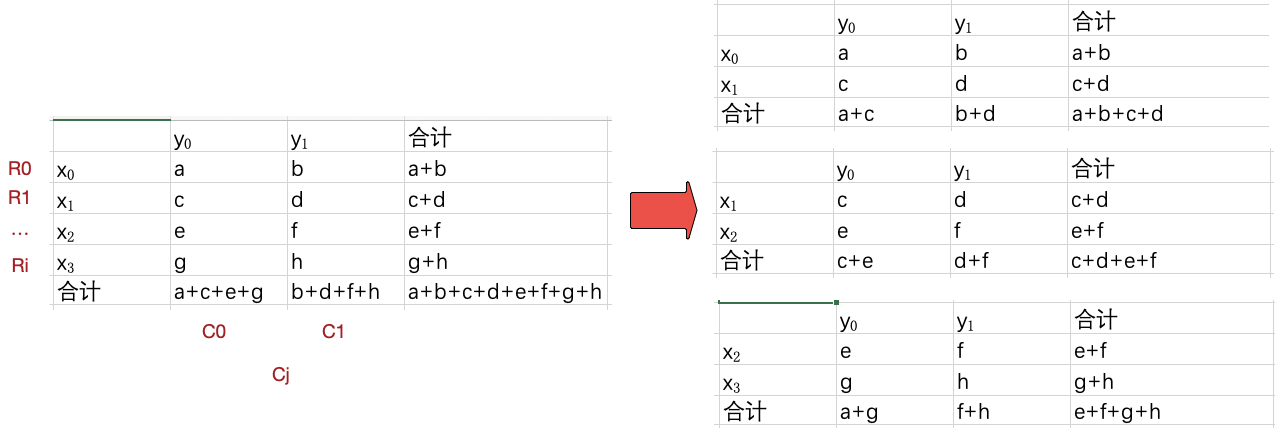卡方分箱中的卡方值是通过计算相邻两项的卡方值得到的。因此，分别计算

(

x

0

,

x

1

)

(x_0,x_1)

，

(

x

1

,

x

2

)

(x_1, x_2)

，

(

x

2

,

x

3

)

(x_2,x_3)

的卡方值，

x

0

x_0

和

x

1

x_1

的卡方值参见上面的介绍。这也正好解释了论文给出的公式中，

i

i

的取值只有两个（每次计算只考虑相邻的两项）。由于对于多分类问题，y的取值不止两个，因此，公式里面j的取值为

[

1

,

k

]

[1,k]

。
在卡方分箱中卡方值计算的图里面，有用红色框框标出来的一句话，这里面有关键的一点解释错了（红色框框标出）。中文解释说，

C

j

/

N

C_j / N

是

j

j

类样本在总体中占的比例。但根据我们前面的介绍，对于

x

0

x_0

和

x

1

x_1

的卡方值，

C

j

/

N

C_j / N

真正表示的是

j

j

类样本在

x

0

x_0

和

x

1

x_1

这两项中占的比例。。
至此，可以给出卡方值计算的代码：
def calc_chi2(count, group1, group2):
"""
根据分组信息（group）计算各分组的卡方值
:param count: DataFrame 待分箱变量各取值的正负样本数
:param group1: list 单个分组信息
:param group2: list 单个分组信息
:return: 该分组的卡方值
"""
count_intv1 = count.loc[count.index.isin(group1)].sum(axis=0).values
count_intv2 = count.loc[count.index.isin(group2)].sum(axis=0).values
count_intv = np.vstack((count_intv1, count_intv2))
# 计算四联表
row_sum = count_intv.sum(axis=1)
col_sum = count_intv.sum(axis=0)
total_sum = count_intv.sum()

# 计算期望样本数
count_exp = np.ones(count_intv.shape) * col_sum / total_sum
count_exp = (count_exp.T * row_sum).T

# 计算卡方值
chi2 = (count_intv - count_exp) ** 2 / count_exp
chi2[count_exp == 0] = 0
return chi2.sum()

chi2_list = [calc_chi2(count, group[idx], group[idx + 1]) for idx in range(len(group) - 1)]


代码实现的时候有一个小trick。对于每个四联表，可以选择用for循环，循环四次，依次计算出每个值对应的期望值

E

i

j

E_{ij}

。但通过简单的矩阵变换，可以将转换为矩阵运算。由卡方值的计算公式可知：

E

i

j

=

[

(

a

+

b

)

(

a

+

c

)

n

(

a

+

b

)

(

b

+

d

)

n

(

a

+

c

)

(

b

+

d

)

n

(

b

+

d

)

(

c

+

d

)

n

]

=

[

a

+

b

c

+

d

]

∗

[

a

+

c

n

b

+

d

n

]

E_{ij} = { \left[\begin{array}{ccc} \frac{(a+b)(a+c)}{n} & \frac{(a+b)(b+d)}{n} \\ \frac{(a+c)(b+d)}{n} & \frac{(b+d)(c+d)}{n} \end{array} \right]}={ \left[\begin{array}{ccc} a+b \\ c+d \end{array} \right]} * { \left[\begin{array}{ccc} \frac{a+c}{n} & \frac{b+d}{n} \end{array} \right]}

即：

E

i

j

=

r

o

w

_

s

u

m

.

T

∗

c

o

l

_

s

u

m

n

=

(

c

o

l

_

s

u

m

n

.

T

∗

r

o

w

_

s

u

m

)

.

T

E_{ij}=row\_sum.T * \frac{col\_sum}{n}= (\frac{col\_sum}{n}.T * row\_sum).T

当你去网上搜索相关的内容时，你会看到很多类似如下的解释和代码。这些代码就是按照中文解释中的思路去计算卡方值，乍一看还挺有道理的。这里很感谢这篇博客Python评分卡建模—卡方分箱，在我犹豫不决的时候，是它让我坚持了自己的想法。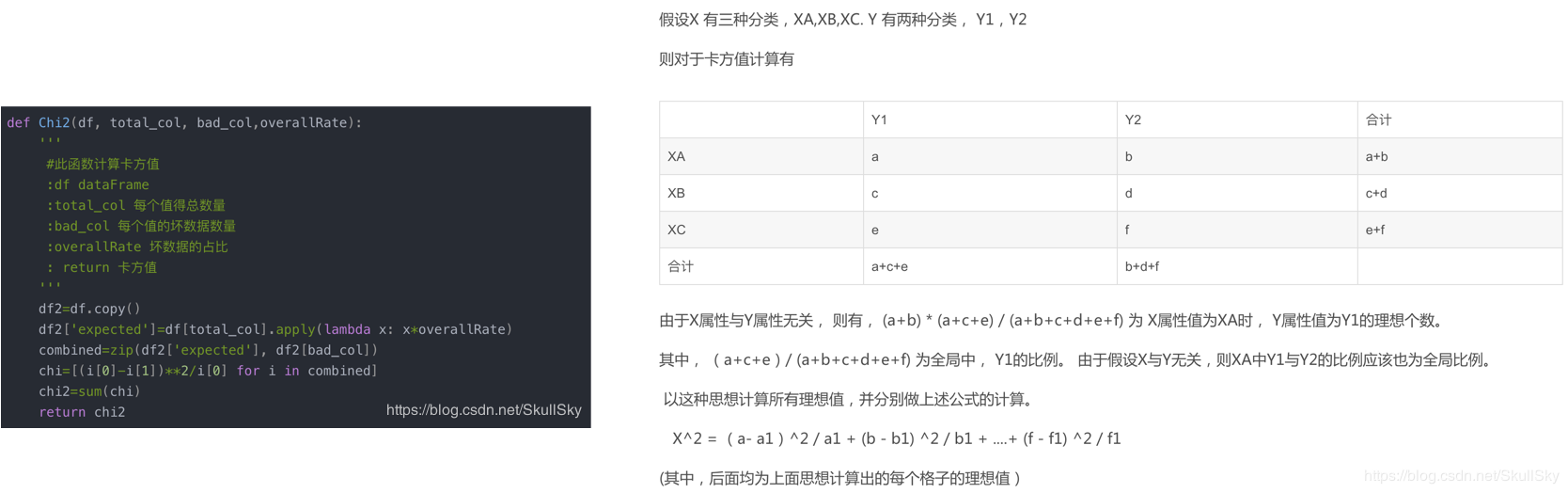2.3 区间合并
这一部分其实没有太多难点，思想很简单，计算得到相邻分组的卡方值后，找到卡方值最小的分组并合。先直接给出初始版本的代码：
def merge_adjacent_intervals(chi2_list, group):
"""
根据卡方值合并卡方值最小的相邻分组
:param chi2_list: list 每个分组的卡方值
:param group: list 分组信息
:return: 合并后的分组信息及卡方值
"""
min_idx = chi2_list.index(min(chi2_list))
# 根据卡方值合并卡方值最小的相邻分组
group[min_idx] = group[min_idx] + group[min_idx+1]
group.remove(group[min_idx+1])
return group

对应的chiMerge代码如下：
def Chi_Merge(count, max_interval=6, sig_level=0.05):
"""
基于ChiMerge的卡方离散化方法
:param count: DataFrame 待分箱变量各取值的正负样本数
:param max_interval: int 最大分箱数量
:param sig_level: 显著性水平(significance level) = 1 - 置信度
:return: 分组信息（group）
"""
print("ChiMerge分箱开始：")
deg_freedom = len(count.columns) - 1 # 自由度(degree of freedom)= y类别数-1
chi2_threshold = chi2.ppf(1 - sig_level, deg_freedom)  # 卡方阈值
group = np.array(count.index).reshape(-1, 1).tolist()  # 分组信息

while len(group) > max_interval:
# 2. 计算相邻分组的卡方值
chi2_list = [calc_chi2(count, group[idx], group[idx + 1]) for idx in range(len(group) - 1)]
print(chi2_list)

# 3. 合并相似分组
if min(chi2_list) >= chi2_threshold:
print("最小卡方值%.3f大于卡方阈值%.3f，分箱合并结束！！！" % (min(chi2_list), chi2_threshold))
break
print("ChiMerge分箱完成！！！")
return group

这里同样可以采用一个小trick，即每次合并区间后，不重新计算整个列表的卡方值，而是动态更新卡方值的数组（chi2_list)。代码如下（大家自行体会，很简单的）：
def merge_adjacent_intervals(count, chi2_list, group):
"""
根据卡方值合并卡方值最小的相邻分组并更新卡方值
:param count: DataFrame 待分箱变量的
:param chi2_list: list 每个分组的卡方值
:param group: list 分组信息
:return: 合并后的分组信息及卡方值
"""
min_idx = chi2_list.index(min(chi2_list))
# 根据卡方值合并卡方值最小的相邻分组
group[min_idx] = group[min_idx] + group[min_idx+1]
group.remove(group[min_idx+1])

# 更新卡方值
if min_idx == 0:
chi2_list.pop(min_idx)
chi2_list[min_idx] = calc_chi2(count, group[min_idx], group[min_idx+1])
elif min_idx == len(group)-1:
chi2_list[min_idx-1] = calc_chi2(count, group[min_idx-1], group[min_idx])
chi2_list.pop(min_idx)
else:
chi2_list[min_idx-1] = calc_chi2(count, group[min_idx-1], group[min_idx])
chi2_list.pop(min_idx)
chi2_list[min_idx] = calc_chi2(count, group[min_idx], group[min_idx+1])
return chi2_list, group

def Chi_Merge1(count, max_interval=6, sig_level=0.05):
"""
基于ChiMerge的卡方离散化方法
:param count: DataFrame 待分箱变量各取值的正负样本数
:param max_interval: int 最大分箱数量
:param sig_level: 显著性水平(significance level) = 1 - 置信度
:return: 分组信息（group）
"""
print("ChiMerge分箱开始：")
deg_freedom = len(count.columns) - 1  # 自由度(degree of freedom)= y类别数-1
chi2_threshold = chi2.ppf(1 - sig_level, deg_freedom)  # 卡方阈值
group = np.array(count.index).reshape(-1, 1).tolist()  # 分组信息

# 2. 计算相邻分组的卡方值
chi2_list = [calc_chi2(count, group[idx], group[idx + 1]) for idx in range(len(group) - 1)]

# 3. 合并相似分组并更新卡方值
while 1:
if min(chi2_list) >= chi2_threshold:
print("最小卡方值%.3f大于卡方阈值%.3f，分箱合并结束！！！" % (min(chi2_list), chi2_threshold))
break
if len(group) <= max_interval:
print("分组长度%s等于指定分组数%s" % (len(group), max_interval))
break
chi2_list, group = merge_adjacent_intervals(count, chi2_list, group)
# print(chi2_list)
print("ChiMerge分箱完成！！！")
return group

2.4 停止条件
卡方分箱的停止条件有如下两种选择： （1）分箱个数等于指定的分箱数目（max_interval）：限制最终的分箱个数结果，每次将样本中具有最小卡方值的 区间与相邻的最小卡方区间进行合并，直到分箱个数达到限制条件为止。 （2）最小卡方值大于卡方阈值（chi2_threshold）：根据自由度和显著性水平得到对应的卡方阈值，如果分箱的各区间最小卡方值小于卡方阈值，则继续合并，直到最小卡方值超过设定阈值为止。 可以两个同时用，也可以只用一个。看实际需求调整即可。
阈值的意义 类别和属性独立时,有90%的可能性,计算得到的卡方值会小于4.6。 大于阈值4.6的卡方值就说明属性和类不是相互独立的，不能合并。如果阈值选的大,区间合并就会进行很多次，离散后的区间数量少、区间大。
需要补充说明的是，
卡方阈值的确定：可以根据显著性水平(significance level) 和自由度（degree of freedom)求得。 自由度一般情况下为类别数减一，如分为3类，自由度为2。则在90%置信度（10%显著水平）下，卡方阈值为4.6。 例如：有3类,自由度为2，则90%置信度(10%显著性水平)下，卡方的值为4.6。 显著性水平的值需要由用户指定，这也是chi2分箱改进的地方。阈值的意义：类别和属性独立时，有90%的可能性，计算得到的卡方值会小于4.6。 大于4.6的卡方值就说明属性和类不是相互独立的，不能合并。阈值越大，区间合并越频繁，离散后的区间数量越少，区间越大。
3. 分箱结果评价
分箱完成后，要对分箱结果进行评价。评分卡模型中最常用的是WOE和IV值，先直接给出代码，后面专门讨论。要注意的一点是，woe和iv值只能针对二分类问题计算。
def calc_IV(count):
"""
计算各分组的WOE值以及IV值
:param count: DataFrame 排好序的各组中正负样本分布
:return: 各分箱的woe和iv值

计算公式：WOE_i = ln{(sum_i / sum_T) / [(size_i - sum_i) / (size_T - sum_T)]}
计算公式：IV_i = [sum_i / sum_T - (size_i - sum_i) / (size_T - sum_T)] * WOE_i
"""
# 计算全体样本中好坏样本的比重
good = (count / count.sum()).values

woe[ind] = 0
print('第%s类负例样本个数为0！！！' % ind)
if 0 in good:
ind = np.where(good == 0)
woe[ind] = 0
print('第%s类正例样本个数为0！！！' % ind)
iv = (good - bad) * woe
return woe, iv

4. 分箱的优点
离散特征的增加和减少都很容易，易于模型的快速迭代；稀疏向量内积乘法运算速度快，计算结果方便存储，容易扩展；列表内容离散化后的特征对异常数据有很强的鲁棒性：比如一个特征是年龄>30是1，否则0。如果特征没有离散化，一个异常数据“年龄300岁”会给模型造成很大的干扰；列表内容逻辑回归属于广义线性模型，表达能力受限；单变量离散化为N个后，每个变量有单独的权重，相当于为模型引入了非线性，能够提升模型表达能力，加大拟合；离散化后可以进行特征交叉，由M+N个变量变为M*N个变量，进一步引入非线性，提升表达能力；列表内容特征离散化后，模型会更稳定，比如如果对用户年龄离散化，20-30作为一个区间，不会因为一个用户年龄长了一岁就变成一个完全不同的人。当然处于区间相邻处的样本会刚好相反，所以怎么划分区间是门学问；特征离散化以后，起到了简化了逻辑回归模型的作用，降低了模型过拟合的风险。 可以将缺失作为独立的一类带入模型。将所有变量变换到相似的尺度上。
5. 代码
关注如下微信公众号，或者微信搜索“机器修行”，回复 离散化 ，获取代码链接。展开全文离散化 离散特征 卡方值
• 统计学，风控建模经常遇到卡方分箱算法ChiMerge。卡方分箱在金融信贷风控领域是逻辑回归评分卡的核心，让分箱具有统计学意义（单调性）。卡方分箱在生物医药领域可以比较两种药物或两组病人是否具有显著区别。但很多...
• ## 卡方分箱算法

千次阅读 2019-06-15 14:18:06
再看这篇文章前，最好先了解卡方分箱算法的原理再来看代码，这样才能有帮助，并且看的时候要从头到尾的看完，不要看一半，有些函数是嵌在其他函数中计算的，明白这点很重要~ 这里主要包括了一些基础函数和主体...
• 卡方分箱介绍及python代码实现 参考这个链接： 一文介绍特征工程里的卡方分箱，附代码实现： https://blog.csdn.net/Pysamlam/article/details/102735480
• 解决的问题：1、实现了二分类的卡方分箱；2、实现了最大分组限定停止条件，和最小阈值限定停止条件；问题，还不太清楚，后续补充。1、自由度k,如何来确定，卡方阈值的自由度为 分箱数-1，显著性水平可以取10%，5%或1...
• ## PART 3.3 风控建模卡方分箱计算篇

千次阅读 热门讨论 2019-03-06 17:17:38
卡方分箱算法 主要包括两个阶段：初始化阶段和自底向上的合并阶段 卡方值计算公式： O =观测频率 E =期望频率 ∑ =总和 X2 =卡方值 卡方分箱excel计算演示 1 对数据进行排序 2 对数据进行分组...风控建模
• 1.分箱 分箱操作就是将连续变量离散化 2.分箱的优点 1.离散化后的特征对异常数据不敏感 2.离散化可以进行特征交叉，提升特征表达能力 ...2.有监督分箱：best-ks分箱和卡方分箱 4.卡方分箱基本思想 ...
• 解决的问题：1、实现了二分类的卡方分箱；2、实现了最大分组限定停止条件，和最小阈值限定停止条件；问题，还不太清楚，后续补充。1、自由度k,如何来确定，卡方阈值的自由度为 分箱数-1，显著性水平可以取10%，5%或1...
• 原标题：Python评分卡建模—卡方分箱今天主要给大家讲讲卡方分箱算法ChiMerge。先给大家介绍一下经常被提到的卡方分布和卡方检验是什么。一、卡方分布 卡方分布(chi-square distribution, χ2-distribution)是概率...
• python_卡方分箱 # 卡方统计量衡量了区间内样本的频数分布与整体样本的频数分布的差异性，在做分箱处理时可以使用两种限制条件： # （1）分箱个数：限制最终的分箱个数结果，每次将样本中具有最小卡方值的区间与...
• ## ChiMerge & 卡方分箱

千次阅读 2019-02-27 18:41:05
# -*- coding: utf-8 -*- """ Created on @author: """ import pandas as pd import numpy as np data = pd.read_csv('iris.csv', sep="\t", na_values=['', '?...# 定义一个卡方分箱（可设置参数置信度水...
• 特征工程之特征分箱：决策树分箱、卡方分箱、bestks以及评价标准python 数据挖掘 机器学习
•  　最近在研究评分卡建模的流程，在特征处理的过程中涉及到分箱这一基本的常用技巧，本文就对分箱中的卡方分箱展开详细介绍。  　分箱就是将连续型的数据离散化，比如年龄这个变量是，可以分箱为0-18，18-30，30-...
• 今天主要给大家讲讲卡方分箱算法ChiMerge。先给大家介绍一下经常被提到的卡方分布和卡方检验是什么。 一、卡方分布 卡方分布(chi-square distribution, χ2-distribution)是概率统计里常用的一种概率分布，也是...
• 今日锦囊特征锦囊：一文介绍特征工程里的卡方分箱，附代码实现今天还是讲一下金融风控的相关知识，上一次我们有讲到，如果我们需要计算变量的IV值，从而判断变量的预测能力强弱，是需要对变量进行离散化的，也就是...
• 风控建模卡方分箱内容详解篇 这是我第一次使用CSDN文章，希望将很多事情做到细致和极致，也希望真的可以给大家带来帮助 客观讲，卡方检验逻辑简单， 基于四个表计算卡方值， 通过自由度，置信度得到该条件下的...风控建模 思维导图
• 卡方分箱原理及实现(Chi Merge 算法) 一. 卡方分布 卡方分布的定义: 若k个独立的随机变量Z1,Z2,…,Zk满足标准正态分布N(0, 1), 则这k个随机变量的平方和: X=∑i=1kZi2 X = \sum_{i=1}^{k}Z_{i}^2 X=i=1∑k​Zi2​ 为...
• 本文针对有一定基础的数据分析人员，专门想了解卡方分箱原理和寻找能直接运行的代码的人员。 分箱是特征工程中常见的操作，也就是将某一个变量划分为多个区间，比如对年龄分箱，1-10岁，10-40岁，40+岁。卡方分箱...python 数据挖掘 机器学习
• 今日锦囊特征锦囊：一文介绍特征工程里的卡方分箱，附代码实现今天还是讲一下金融风控的相关知识，上一次我们有讲到，如果我们需要计算变量的IV值，从而判断变量的预测能力强弱，是需要对变量进行离...数据分析 统计学 python 人工智能 大数据
• 1.数据源文件 ...提取码: 2s3k 2.处理连续没有正样本或负样本的区间 import pandas as pd import numpy as np ...# 定义一个卡方分箱（可设置参数置信度水平与箱的个数）停止条件为大于置信水平且小于bin的数目 def Ch
• ppd_score 拍拍贷的一个贷款预测比赛，里面用到了信用评分卡相关知识，比如WOE，IV值，卡方分箱，KS值等Python
• ## 【有监督分箱】方法一：卡方分箱

万次阅读 多人点赞 2018-05-06 19:01:25
何谓分箱，简单地说，分箱就是将连续变量离散化，将多状态的离散变量合并成少状态。 3.分箱的用处 离散特征的增加和减少都很容易，易于模型的快速迭代； 稀疏向量内积乘法运算速...
• 初次接触变量分箱是在做评分卡模型的时候，SAS软件里有一段宏可以直接进行连续变量的最优分箱，但如果搬到Python的话，又如何实现同样或者说类似的操作呢，今天就在这里简单介绍一个办法——卡方分箱算法。......